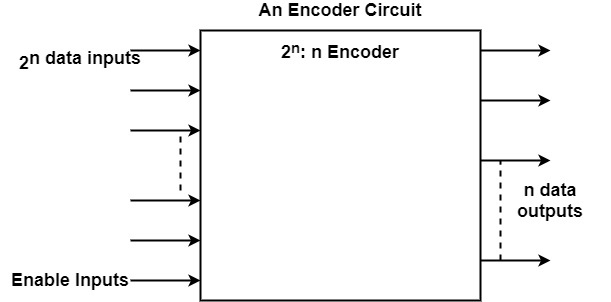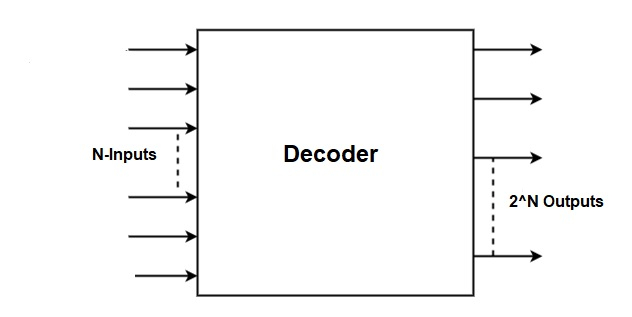# What is the difference between Encoder and Decoder in Computer architecture?

## Encoder

A digital circuit that executes the inverse services of a decoder is known as an encoder. It has 2n input lines and n output lines. In an encoder, the output lines create the binary code equivalent to the input value. The figure displays the general architecture of an encoder circuit.## Decoder

The decoder is an electronic device that can modify a digital signal to an analog signal. It enables a single input line and creates multiple output lines. The decoders are used in several communication projects that are connected between two devices. The decoder enables N- inputs and creates 2 power N-numbers of outputs. For instance, if it can provide 2 inputs that will make 4 outputs by using 4 by 2 decoder.

Some decoders are established with NAND instead of AND gates. In this term, all decoder outputs will be 1’s except the one equivalent to the input code which will be 0. 2-to-4 line decoder with an enable input generated with NAND gates. The circuit works with complemented outputs and allows input E’, which is also integrated to connect the outputs of the decoder NAND gate. The decoder is allowed when E’ is similar to zero.

There is only one output that can be similar to zero at any given time, all several outputs being similar to one. The outputs define the minterm choose by the inputs A1 and A0. The circuit is disabled when E’ is similar to one, unconcerned of the values of the additional two inputs. If the circuit is disabled, then none of the outputs are similar to zero.Let us see the comparison between Encoder and Decoder

EncoderDecoder
An encoder is a digital circuit that implements the reverse operation of a decoder. An encoder has 2n input lines and n output lines.
A decoder is a combinational circuit that modifies binary data from n input lines to a maximum of 2n unique output lines.
An encoder creates the binary code corresponding to the input activated.
A decoder gets a set of binary inputs and activates only the output that complements that input number.
An example of the encoder is the Octal to Binary encoder.
An example of the decoder is the Binary to Octal encoder.
The encoder creates coded data bits as its output that is delivered to the decoder.
A decoder supports an active output signal in feedback to the coded data bits.
An encoder is completely straightforward.
A decoder is complex as it requires understanding the coding structure of the encoder.
The encoder is set up at the sending end.
The decoder circuit is set up at the receiving side.